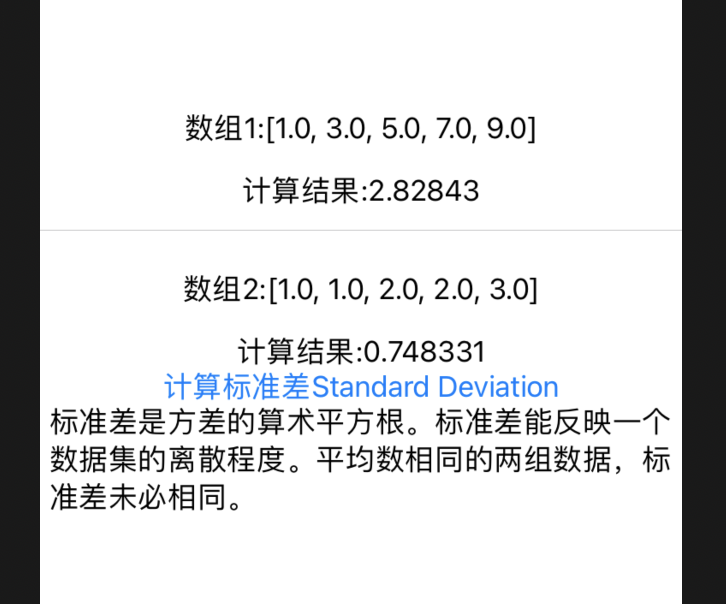www.b2bchain.cn

# 本文价值与收获

## 看完本文后，您将能够作出下面的界面# SwiftUI 计算数组标准差Standard Deviation

“`
import SwiftUI

struct ContentView: View {
@State var data1 = [1.0,3.0,5.0,7.0,9.0]
@State var rs1 = 0.0
@State var data2 = [1.0,1.0,2.0,2.0,3.0]
@State var rs2 = 0.0
var body: some View {
VStack{
Text(“计算结果:(self.rs1,specifier: “%g”)”)
Divider()
Text(“计算结果:(self.rs2,specifier: “%g”)”)

``        Button("计算标准差Standard Deviation"){             self.rs1 = self.data1.std             self.rs2 = self.data2.std         }         Text("标准差是方差的算术平方根。标准差能反映一个数据集的离散程度。平均数相同的两组数据，标准差未必相同。")     } }``

}

# 本文价值与收获

## 看完本文后，您将能够作出下面的界面# SwiftUI 计算数组标准差Standard Deviation

“`
import SwiftUI

struct ContentView: View {
@State var data1 = [1.0,3.0,5.0,7.0,9.0]
@State var rs1 = 0.0
@State var data2 = [1.0,1.0,2.0,2.0,3.0]
@State var rs2 = 0.0
var body: some View {
VStack{
Text(“计算结果:(self.rs1,specifier: “%g”)”)
Divider()
Text(“计算结果:(self.rs2,specifier: “%g”)”)

``        Button("计算标准差Standard Deviation"){             self.rs1 = self.data1.std             self.rs2 = self.data2.std         }         Text("标准差是方差的算术平方根。标准差能反映一个数据集的离散程度。平均数相同的两组数据，标准差未必相同。")     } }``

}

# 本文价值与收获

## 看完本文后，您将能够作出下面的界面# SwiftUI 计算数组标准差Standard Deviation

“`
import SwiftUI

struct ContentView: View {
@State var data1 = [1.0,3.0,5.0,7.0,9.0]
@State var rs1 = 0.0
@State var data2 = [1.0,1.0,2.0,2.0,3.0]
@State var rs2 = 0.0
var body: some View {
VStack{
Text(“计算结果:(self.rs1,specifier: “%g”)”)
Divider()
Text(“计算结果:(self.rs2,specifier: “%g”)”)

``        Button("计算标准差Standard Deviation"){             self.rs1 = self.data1.std             self.rs2 = self.data2.std         }         Text("标准差是方差的算术平方根。标准差能反映一个数据集的离散程度。平均数相同的两组数据，标准差未必相同。")     } }``

}

• 微信咨询
• 回顶